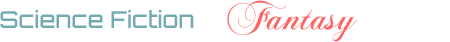10

## Please login or register to vote for this query.

(click on this box to dismiss)

This shows how much of the users reputation came from questions as opposed to answers or edits, bounties, etc. Can take 60 seconds to run.Q&A for science fiction and fantasy enthusiasts

``````-- Reputation breakdown: Question, versus answer, versus other
-- This shows how much of the users reputation came from questions as opposed
-- to answers or edits, bounties, etc. Can take 60 seconds to run.

DECLARE @UserId         INT = ##UserId##;
DECLARE @TotalRep       INT,
@Q_Accepted     INT,
@A_Accepted     INT,
@BountyGive     INT,
@BountyGot      INT,
@DwnVoteGiven   INT,
@MiscEdits      INT,
@WikiEdits      INT,
@BonusOther     INT,
@UncappedTotal  INT;
DECLARE @LatestDate     DATETIME;

SELECT  @TotalRep       = COALESCE (Reputation, 0) + 1,    -- Base of 1 not in total!?
@DwnVoteGiven   = COALESCE (-1 * DownVotes / 2, 0) -- estimate
FROM    Users
WHERE   Id              = @UserId

SELECT      @Q_Accepted         = SUM (2)
FROM        Posts               p
INNER JOIN  Posts               a
ON          p.AcceptedAnswerId  = a.Id
WHERE       p.OwnerUserId       = @UserId
AND         a.OwnerUserId      != @UserId

SELECT      @WikiEdits          = SUM (2)
FROM        PostHistory         ph
INNER JOIN  Posts               p
ON          ph.PostId           = p.Id
WHERE       p.PostTypeId        = 3
AND         IsNull (ph.UserId, -1) = ##UserId##;

WITH    DailyRepCTE (Reputation, CreationDate)
AS (
SELECT
SUM (   CASE p.PostTypeId
WHEN 1  THEN
CASE v.VoteTypeId
WHEN    2 THEN   5
WHEN    3 THEN  -2
ELSE             0
END
WHEN 2  THEN
CASE v.VoteTypeId
WHEN    2 THEN  10
WHEN    3 THEN  -2
ELSE             0
END
ELSE    0
END
)
AS [Reputation]
, v.CreationDate
FROM        Posts p
LEFT JOIN   Votes v
ON          p.Id                    = v.PostId
WHERE       p.OwnerUserId           = @UserId
AND         p.CommunityOwnedDate    IS NULL
GROUP BY    v.CreationDate
)
SELECT      @RepCapAdjust = SUM (Reputation - 200)
FROM        DailyRepCTE
WHERE       Reputation  > 200

SELECT
@Q_UpVotes  = SUM ( CASE p.PostTypeId
WHEN 1  THEN
CASE v.VoteTypeId
WHEN    2 THEN   5
ELSE             0
END
ELSE    0
END
),
@Q_DwnVotes = SUM ( CASE p.PostTypeId
WHEN 1  THEN
CASE v.VoteTypeId
WHEN    3 THEN  -2
ELSE             0
END
ELSE    0
END
),
@A_UpVotes  = SUM ( CASE p.PostTypeId
WHEN 2  THEN
CASE v.VoteTypeId
WHEN    2 THEN  10
ELSE             0
END
ELSE    0
END
),
@A_DwnVotes = SUM ( CASE p.PostTypeId
WHEN 2  THEN
CASE v.VoteTypeId
WHEN    3 THEN  -2
ELSE             0
END
ELSE    0
END
),
@A_Accepted = SUM ( CASE p.PostTypeId
WHEN 2  THEN
CASE v.VoteTypeId
WHEN    1 THEN  15
ELSE             0
END
ELSE    0
END
),
@BountyGive = SUM ( CASE v.VoteTypeId   -- These are not in DB!!
WHEN 8  THEN    v.BountyAmount
ELSE            0
END
),
@BountyGot  = SUM ( CASE v.VoteTypeId
WHEN 9  THEN    v.BountyAmount
ELSE            0
END
),
@MiscEdits  = SUM ( CASE v.VoteTypeId
WHEN 16 THEN    2
ELSE            0
END
),
@LatestDate = MAX (v.CreationDate)
FROM        Posts p
LEFT JOIN   Votes v
ON          p.Id                    = v.PostId
WHERE       p.OwnerUserId           = @UserId
AND         p.CommunityOwnedDate    IS NULL

SET @Q_Accepted     = COALESCE (@Q_Accepted,    0);
SET @A_Accepted     = COALESCE (@A_Accepted,    0);
SET @BountyGive     = COALESCE (@BountyGive,    0);
SET @BountyGot      = COALESCE (@BountyGot,     0);
SET @MiscEdits      = COALESCE (@MiscEdits,     0)  +  COALESCE (@WikiEdits, 0);

SET @UncappedTotal  = @TotalRep + @RepCapAdjust
SET @BonusOther     = @UncappedTotal
- @Q_Accepted
- @A_Accepted
- @BountyGive
- @BountyGot
- @DwnVoteGiven
- @MiscEdits
;

DECLARE @Results TABLE (
item        VARCHAR(60),
Reputation  VARCHAR(60),
[Percent]   FLOAT
)

INSERT INTO
@Results (item, Reputation, [Percent])
VALUES
('Total (capped)       ', CAST (@TotalRep      AS VARCHAR(60) ), ROUND (@TotalRep      * 100.0 / @UncappedTotal, 1) ),
('Total (uncapped)     ', CAST (@UncappedTotal AS VARCHAR(60) ), 100.0                                              ),
('Quest Accepted       ', CAST (@Q_Accepted    AS VARCHAR(60) ), ROUND (@Q_Accepted    * 100.0 / @UncappedTotal, 1) ),
('Quest Upvotes        ', CAST (@Q_UpVotes     AS VARCHAR(60) ), ROUND (@Q_UpVotes     * 100.0 / @UncappedTotal, 1) ),
('Quest Downvotes      ', CAST (@Q_DwnVotes    AS VARCHAR(60) ), ROUND (@Q_DwnVotes    * 100.0 / @UncappedTotal, 1) ),
('Ans Accepted         ', CAST (@A_Accepted    AS VARCHAR(60) ), ROUND (@A_Accepted    * 100.0 / @UncappedTotal, 1) ),
('Ans Upvotes          ', CAST (@A_UpVotes     AS VARCHAR(60) ), ROUND (@A_UpVotes     * 100.0 / @UncappedTotal, 1) ),
('Ans Downvotes        ', CAST (@A_DwnVotes    AS VARCHAR(60) ), ROUND (@A_DwnVotes    * 100.0 / @UncappedTotal, 1) ),
--  ('Bounties Given       ', CAST (@BountyGive    AS VARCHAR(60) ), ROUND (@BountyGive    * 100.0 / @UncappedTotal, 1) ),
('Bounties Given       ', 'Not in Data Explorer!?',              ROUND (@BountyGive    * 100.0 / @UncappedTotal, 1) ),
('Bounties Received    ', CAST (@BountyGot     AS VARCHAR(60) ), ROUND (@BountyGot     * 100.0 / @UncappedTotal, 1) ),
('Downvotes Given (est)', CAST (@DwnVoteGiven  AS VARCHAR(60) ), ROUND (@DwnVoteGiven  * 100.0 / @UncappedTotal, 1) ),
('Misc Edits           ', CAST (@MiscEdits     AS VARCHAR(60) ), ROUND (@MiscEdits     * 100.0 / @UncappedTotal, 1) ),
('Bonus / Other        ', CAST (@BonusOther    AS VARCHAR(60) ), ROUND (@BonusOther    * 100.0 / @UncappedTotal, 1) ),
('Most Recent Date     ', CONVERT (VARCHAR(60), @LatestDate, 101),   null                                      )

SELECT * FROM @Results``````

### Enter Parameters

Options:
-Hold tight while we fetch your results
:records returned in :time ms:cached